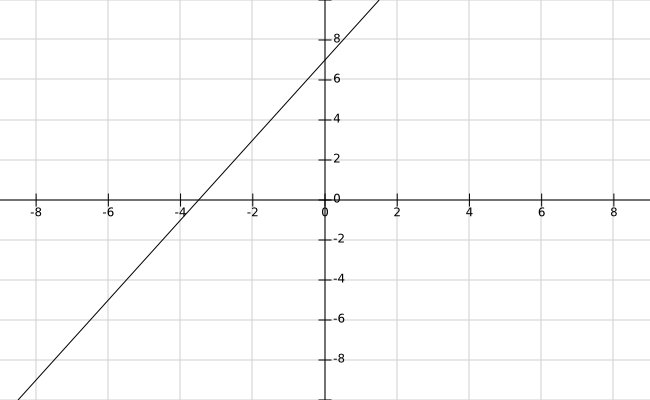# Relations and FunctionsIn mathematics, a relation is a connection between two distinct pieces of data or variables. For example, student name and ID number would be a relation commonly found at a school. What this means is that you can refer to a student by there name and get their ID number and vice versa.  These two pieces of information are connected and refer to each other. Another term for relation is ordered pair, however, this is more commonly use for coordinate graphing. Below is an example of several student names and ID numbers

Student Name (x values) ID Number (y values)
Jill Smith 12345
Eve Jackson 54321
John Doe 24681

Table 1

Two other pieces of information to know are domain and range. The domain represents all x values. In our table above the student names are the x values (Jill Smith, Eve Jackson, John Doe). The range is all of the y-values, THese are represented by ID number in the table above (12345, 54321, 24681).

The table above is nice and neat. However, sometimes the information is not organized into neat rows but is scrambled with the names and ID numbers not lining up. Below is the same information as the table 1 but the ID numbers are scrambled. The arrows tell who the ID number belongs to who.  This is known as mapping.

Student Name ID Number
Jill Smith ↘ 24681
Eve Jackson→ 54321
John Doe↗ 12345

If we find the ordered pair, domain and range it would be as follows.

• Ordered pair = {(Jill Smith, 123450, (Eva Jackson, 54321), (John Doe,  24681)}
• domain = {Jill Smith, Eva Jackson, John Doe}
• Range = {24681, 54321, 12345}

Understanding Functions

A function is a specific type of relation. What a function does is assigns to each element in a domain. Below is an example of a function

# f(x) = 2x + 7

Functions are frequently written to look the same as an equation  as shown below

# y = 2x + 7

PLugging in different values of x in your function will provide you with a y as shown belowHere our x-value is 2 and the y-value is 11.

Of course, you can graph function as any other linear equation. Below is a visual.Conclusion

This post explained the power of relations and functions. Relations are critical in computer science in particular relational databases. In addition., Functions are a bedrock in statistics and other forms of math. Therefore it is critical to understand these basic concepts of algebra.